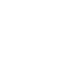# SAT Question of the Day (ACT too!): Nov. 18, 2013

If you are reading this in an email you received from me, do not click the link to sat.collegeboard.org below. Use the link to my website that is farther down on the email.

If you are seeing this in my blog, do the SAT Question of the Day by clicking on this link:

http://sat.collegeboard.org/practice/sat-question-of-the-day?questionId=20131118&oq=1 (This link takes you to today’s question. If you use my archive, you will see the question related to my SAT explanation for that date.)

The answer is A.  Wow! There are very few students getting this one right; so, it is much harder than it looks.  Only 35% of the students who are trying this question are getting it right.  Considering that 20% would get it correct just by randomly guessing, that’s not very many.

Let’s see what we can do with it.  First, the test writer’s explanation is a good “math teacher” way to do the question and you should take a look at it.

Second, there’s another easier, quicker, and more efficient way to do the questions that works for most students.  It’s the “Wizard’s Way.”  I have a few names for the strategy: 1) change the algebra to arithmetic, 2) change the abstract to the concrete, or 3) substitute numbers for variables.  The strategy is pretty simple and is based on common sense.  Oftentimes, the test writers don’t give you numbers but rather give you a variable and that is what causes the question to be confusing.  In this case, they give you d.   Wouldn’t it have been easier if instead of d, they would have given you an actual number?  Sure it would.  So, let’s just make one up because no matter how much you make d, the answer will be the same because “the world of math is a world of patterns.”  I used 1 instead of d.  That means 10 pounds of apples cost \$1 or 10 cents for one pound.  Then they tell me a pound is six apples so a dozen apples have to cost 20 cents.  Which answer is 20 cents?  Answer A is 20 times 1–that’s 20; so, it’s the right answer.  Let’s try the other answers just to confirm we are correct.  Answer B is 50 x 1/3.  That’s not 20.  C is 5 times 1 which isn’t 20.  D is 5 times 1 divided by 3; that one is wrong as well.  Finally, E is 1/20 and that one is also wrong.  Is this strategy easier for you?  Whenever you see variables in a question like this, you can change the algebra to arithmetic and make the question much more concrete.  Usually students find doing questions without numbers much easier when they convert the variables to numbers.  Give it a try and do a little practice.  It should help.

Let’s see what the ACT folks have for us this morning.

The answer is F.  Yep, you sure could use the basic trig identity that the test writer uses in their “math teacher” explanation and there’s nothing wrong with that.

However, what do you do if you can’t remember that on test day?  Use much simpler trig knowledge in combination with the Pythagorean Theorem.  The sin is defined as the opposite/hypotenuse.  So the opposite side is 10 and the hypotenuse is 13.  Pythagoras tells us that 102 + x2 = 132.  So, 100 + x2 is 169.  Doing a little algebra gets you x2 is 69.  That means the adjacent side is the square root of 69.  The cos is adjacent/hypotenuse: √69/13, Answer F.  Boom!  All done!

Which approach works best for you?  Remember there’s always more than one way to do the math questions on the ACT and SAT.  Practicing will help you figure out which strategies are going to raise your score on test day.

What are you going to do this week to make it a great week?  Set your goal now and get it done!

The SAT & ACT Wizard